﻿ Beam Deflection Tables | MechaniCalc

The tables below give equations for the deflection, slope, shear, and moment along straight beams for different end conditions and loadings. You can find comprehensive tables in references such as Gere, Lindeburg, and Shigley. However, the tables below cover most of the common cases.

For information on beam deflection, see our reference on stresses and deflections in beams.

### Cantilever Beams

Cantilever, End LoadDeflection: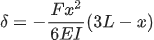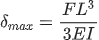@ x = L

Slope: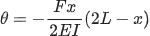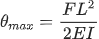@ x = L

Shear:

 V = +F

Moment:

 M = −F (L − x)
 Mmax = −FL @ x = 0
Cantilever, Intermediate Load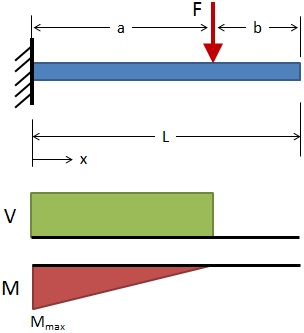Deflection: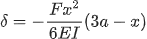( 0 ≤ x ≤ a )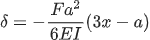( a ≤ x ≤ L )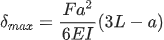@ x = L

Slope: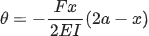( 0 ≤ x ≤ a )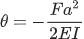( a ≤ x ≤ L )

Shear:

 V = +F ( 0 ≤ x ≤ a ) V = 0 ( a ≤ x ≤ L )

Moment:

 M = −F (a − x) ( 0 ≤ x ≤ a ) M = 0 ( a ≤ x ≤ L )
Cantilever, Uniform Distributed Load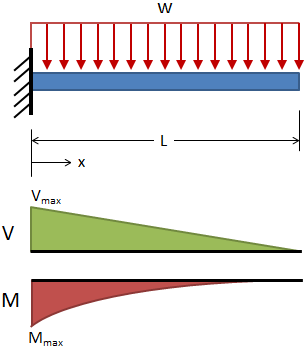Deflection: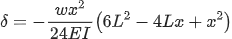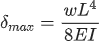@ x = L

Slope: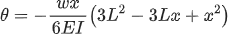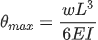@ x = L

Shear:

 V = +w (L − x)
 Vmax = +wL @ x = 0

Moment:

 M = −w (L − x)2 / 2
 Mmax = −wL2 / 2 @ x = 0
Cantilever, Triangular Distributed Load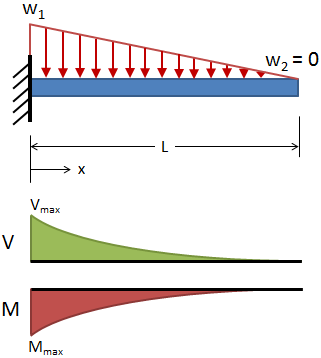Deflection: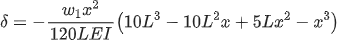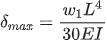@ x = L

Slope: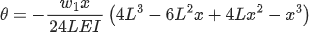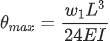@ x = L

Shear:

 Vmax = +w1L / 2 @ x = 0

Moment:

 Mmax = −w1L2 / 6 @ x = 0
Cantilever, End Moment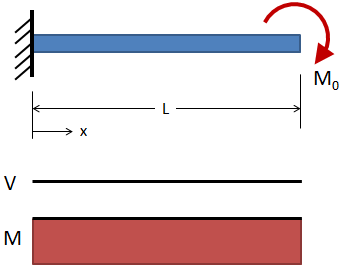Deflection: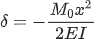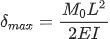@ x = L

Slope: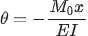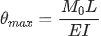@ x = L

Shear:

 V = 0

Moment:

 M = −M0

### Simply Supported Beams

Simply Supported, Intermediate Load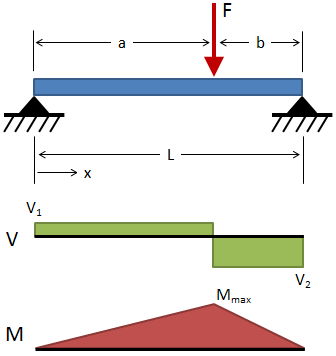Deflection: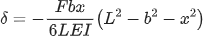( 0 ≤ x ≤ a )

For a ≥ b: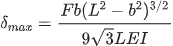@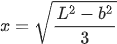Slope: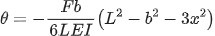( 0 ≤ x ≤ a )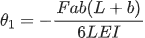@ x = 0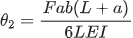@ x = L

Shear:

 V1 = +Fb / L ( 0 ≤ x ≤ a ) V2 = −Fa / L ( a ≤ x ≤ L )

Moment:

 Mmax = +Fab / L @ x = a
Simply Supported, Center LoadDeflection: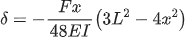( 0 ≤ x ≤ L/2 )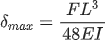@ x = L/2

Slope: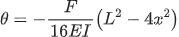( 0 ≤ x ≤ L/2 )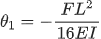@ x = 0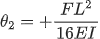@ x = L

Shear:

 V1 = +F / 2 ( 0 ≤ x ≤ L/2 ) V2 = −F / 2 ( L/2 ≤ x ≤ L )

Moment:

 Mmax = FL / 4 @ x = L/2
Simply Supported, 2 Loads at Equal Distances from Supports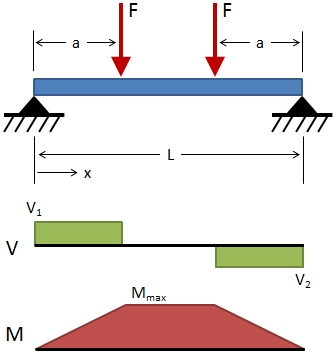Deflection: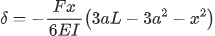( 0 ≤ x ≤ a )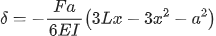( a ≤ x ≤ L − a )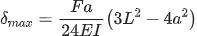@ x = L/2

Slope: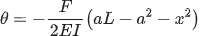( 0 ≤ x ≤ a )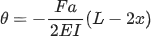( a ≤ x ≤ L − a )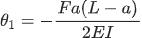@ x = 0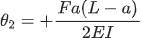@ x = L

Shear:

 V1 = +F ( 0 ≤ x ≤ a ) V2 = −F ( L − a ≤ x ≤ L )

Moment:

 Mmax = Fa ( a ≤ x ≤ L − a )
Simply Supported, Uniform Distributed Load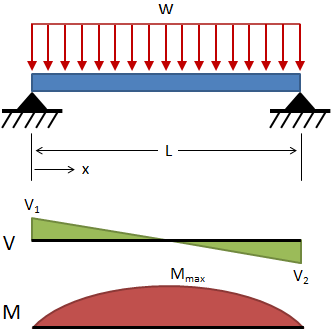Deflection: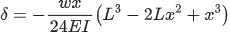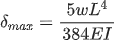@ x = L/2

Slope: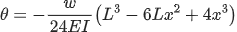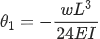@ x = 0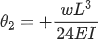@ x = L

Shear:

 V = w (L/2 − x)
 V1 = +wL / 2 @ x = 0 V2 = −wL / 2 @ x = L

Moment:

 Mmax = wL2 / 8 @ x = L/2
Simply Supported, Moment at Each Support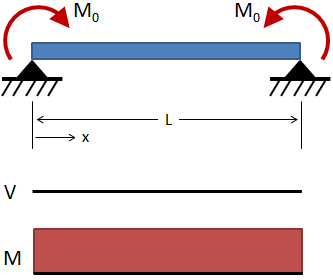Deflection: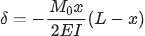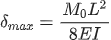@ x = L/2

Slope: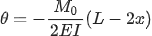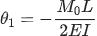@ x = 0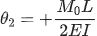@ x = L

Shear:

 V = 0

Moment:

 M = M0
Simply Supported, Moment at One Support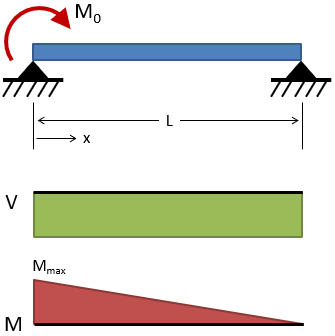Deflection: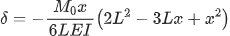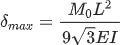@ x = L (1 − √3/3)

Slope: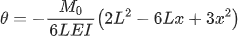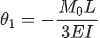@ x = 0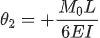@ x = L

Shear:

 V = −M0 / L

Moment:

 Mmax = M0 @ x = 0
Simply Supported, Center MomentDeflection: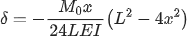( 0 ≤ x ≤ L/2 )

Slope: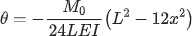( 0 ≤ x ≤ L/2 )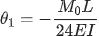@ x = 0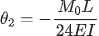@ x = L

Shear:

 V = +M0 / L

Moment:

 M = M0x / L ( 0 ≤ x ≤ L/2 ) Mmax = M0 / 2 @ x = L/2

### Fixed-Fixed Beams

Fixed-Fixed, Center Load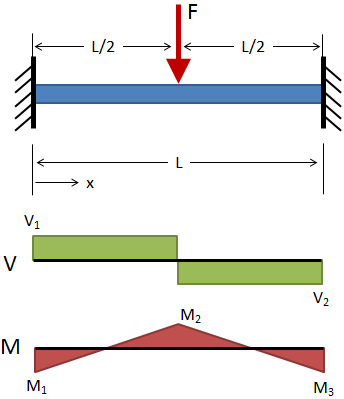Deflection: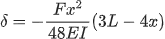( 0 ≤ x ≤ L/2 )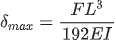@ x = L/2

Shear:

 V1 = +F / 2 ( 0 ≤ x ≤ L/2 ) V2 = −F / 2 ( L/2 ≤ x ≤ L )

Moment:

 M = F (4x − L) / 8 ( 0 ≤ x ≤ L/2 )
 M1 = M3 = −FL / 8 @ x = 0 & x = L M2 = +FL / 8 @ x = L/2
Fixed-Fixed, Uniform Distributed Load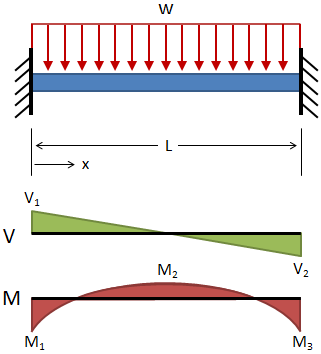Deflection: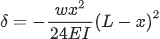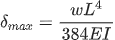@ x = L/2

Shear:

 V = w (L/2 − x)
 V1 = +wL / 2 @ x = 0 V2 = −wL / 2 @ x = L

Moment:

 M = w (6Lx − 6x2 − L2) / 12
 M1 = M3 = −wL2 / 12 @ x = 0 & x = L M2 = wL2 / 24 @ x = L/2

#### Need a Beam Calculator?

Check out our beam calculator based on the methodology described here.

• Calculates stresses and deflections in straight beams
• Builds shear and moment diagrams
• Can specify any configuration of constraints, concentrated forces, and distributed forces